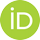# Overview

Doctoral Prize Fellow at the Department of Mathematical Sciences at Loughborough Universityorcid.org/0000-0002-6982-4505Google ScholarMathstodon.xyzYoutube Channel

I started my studies in mathematics at the KU Leuven in Belgium, my native country. After obtaining a bachelor’s degree there in 2012 I moved to Berlin to enter the graduate program of Berlin Mathematical school. I joined the geometry and mathematical physics research group at the Technische Universität Berlin and obtained a master’s degree (2014) and a doctorate (2018). (The respective theses can be found on the publications page.) In 2020 and 2021 I was a DFG Research Fellow at the University of Leeds, UK. In 2022 I started a Doctoral Prize Fellowship at Loughborough University.

## Research Interests

My research is centered around Lagrangian (i.e. variational) dynamical systems and can be broadly divided in two areas. One area involves geometric numerical integration of Lagrangian systems, that is variational integrators. The second part deals with integrable systems. While integrable ODEs or hierarchies of PDEs are usually described in a Hamiltonian framework, but allow a beautiful variational principle too.

### Integrable systems

• Variational principles for continuous and discrete integrable systems: pluri-Lagrangian or Lagrangian multiform theory
• Hierarchies of integrable differential equations as continuum limits of fully discrete equations

(You can find a layman’s introduction to integrable systems in this blog post; and an introduction assuming some mathematical background in these slides)

### Geometric numerical integration

• Backward error analysis for variational integrators
• Discretization of contact Hamiltonian systems

(You can find a light-hearted introduction to geometric numerical integration, assuming some mathematical background, in these slides: A picture book of geometric numerical integration)

### More details

See the list of my publications and the list of talks I’ve given